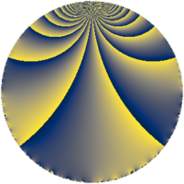Properties

 Label 4560.2.a.bpLevel $4560$ Weight $2$ Character orbit 4560.a Self dual yes Analytic conductor $36.412$ Analytic rank $0$ Dimension $2$ CM no Inner twists $1$

Related objects

Newspace parameters

 Level: $$N$$ $$=$$ $$4560 = 2^{4} \cdot 3 \cdot 5 \cdot 19$$ Weight: $$k$$ $$=$$ $$2$$ Character orbit: $$[\chi]$$ $$=$$ 4560.a (trivial)

Newform invariants

 Self dual: yes Analytic conductor: $$36.4117833217$$ Analytic rank: $$0$$ Dimension: $$2$$ Coefficient field: $$\Q(\sqrt{7})$$ Defining polynomial: $$x^{2} - 7$$ Coefficient ring: $$\Z[a_1, \ldots, a_{7}]$$ Coefficient ring index: $$1$$ Twist minimal: no (minimal twist has level 2280) Fricke sign: $$-1$$ Sato-Tate group: $\mathrm{SU}(2)$

$q$-expansion

Coefficients of the $$q$$-expansion are expressed in terms of $$\beta = \sqrt{7}$$. We also show the integral $$q$$-expansion of the trace form.

 $$f(q)$$ $$=$$ $$q + q^{3} + q^{5} + ( 1 + \beta ) q^{7} + q^{9} +O(q^{10})$$ $$q + q^{3} + q^{5} + ( 1 + \beta ) q^{7} + q^{9} + ( 3 + \beta ) q^{11} + ( 1 - \beta ) q^{13} + q^{15} + q^{19} + ( 1 + \beta ) q^{21} -2 q^{23} + q^{25} + q^{27} + ( 7 - \beta ) q^{29} + 2 q^{31} + ( 3 + \beta ) q^{33} + ( 1 + \beta ) q^{35} + ( 1 + 3 \beta ) q^{37} + ( 1 - \beta ) q^{39} + ( -1 - \beta ) q^{41} + ( -3 - 3 \beta ) q^{43} + q^{45} -6 q^{47} + ( 1 + 2 \beta ) q^{49} + 4 q^{53} + ( 3 + \beta ) q^{55} + q^{57} + ( 2 + 2 \beta ) q^{59} + ( 6 - 2 \beta ) q^{61} + ( 1 + \beta ) q^{63} + ( 1 - \beta ) q^{65} -4 q^{67} -2 q^{69} + ( -2 - 2 \beta ) q^{71} -2 q^{73} + q^{75} + ( 10 + 4 \beta ) q^{77} + 8 q^{79} + q^{81} -6 \beta q^{83} + ( 7 - \beta ) q^{87} + ( 1 + 5 \beta ) q^{89} -6 q^{91} + 2 q^{93} + q^{95} + ( 5 - 5 \beta ) q^{97} + ( 3 + \beta ) q^{99} +O(q^{100})$$ $$\operatorname{Tr}(f)(q)$$ $$=$$ $$2 q + 2 q^{3} + 2 q^{5} + 2 q^{7} + 2 q^{9} + O(q^{10})$$ $$2 q + 2 q^{3} + 2 q^{5} + 2 q^{7} + 2 q^{9} + 6 q^{11} + 2 q^{13} + 2 q^{15} + 2 q^{19} + 2 q^{21} - 4 q^{23} + 2 q^{25} + 2 q^{27} + 14 q^{29} + 4 q^{31} + 6 q^{33} + 2 q^{35} + 2 q^{37} + 2 q^{39} - 2 q^{41} - 6 q^{43} + 2 q^{45} - 12 q^{47} + 2 q^{49} + 8 q^{53} + 6 q^{55} + 2 q^{57} + 4 q^{59} + 12 q^{61} + 2 q^{63} + 2 q^{65} - 8 q^{67} - 4 q^{69} - 4 q^{71} - 4 q^{73} + 2 q^{75} + 20 q^{77} + 16 q^{79} + 2 q^{81} + 14 q^{87} + 2 q^{89} - 12 q^{91} + 4 q^{93} + 2 q^{95} + 10 q^{97} + 6 q^{99} + O(q^{100})$$

Embeddings

For each embedding $$\iota_m$$ of the coefficient field, the values $$\iota_m(a_n)$$ are shown below.

For more information on an embedded modular form you can click on its label.

Label $$\iota_m(\nu)$$ $$a_{2}$$ $$a_{3}$$ $$a_{4}$$ $$a_{5}$$ $$a_{6}$$ $$a_{7}$$ $$a_{8}$$ $$a_{9}$$ $$a_{10}$$
1.1
 −2.64575 2.64575
0 1.00000 0 1.00000 0 −1.64575 0 1.00000 0
1.2 0 1.00000 0 1.00000 0 3.64575 0 1.00000 0
 $$n$$: e.g. 2-40 or 990-1000 Significant digits: Format: Complex embeddings Normalized embeddings Satake parameters Satake angles

Atkin-Lehner signs

$$p$$ Sign
$$2$$ $$1$$
$$3$$ $$-1$$
$$5$$ $$-1$$
$$19$$ $$-1$$

Inner twists

This newform does not admit any (nontrivial) inner twists.

Twists

By twisting character orbit
Char Parity Ord Mult Type Twist Min Dim
1.a even 1 1 trivial 4560.2.a.bp 2
4.b odd 2 1 2280.2.a.n 2
12.b even 2 1 6840.2.a.w 2

By twisted newform orbit
Twist Min Dim Char Parity Ord Mult Type
2280.2.a.n 2 4.b odd 2 1
4560.2.a.bp 2 1.a even 1 1 trivial
6840.2.a.w 2 12.b even 2 1

Hecke kernels

This newform subspace can be constructed as the intersection of the kernels of the following linear operators acting on $$S_{2}^{\mathrm{new}}(\Gamma_0(4560))$$:

 $$T_{7}^{2} - 2 T_{7} - 6$$ $$T_{11}^{2} - 6 T_{11} + 2$$ $$T_{13}^{2} - 2 T_{13} - 6$$ $$T_{17}$$

Hecke characteristic polynomials

$p$ $F_p(T)$
$2$ $$T^{2}$$
$3$ $$( -1 + T )^{2}$$
$5$ $$( -1 + T )^{2}$$
$7$ $$-6 - 2 T + T^{2}$$
$11$ $$2 - 6 T + T^{2}$$
$13$ $$-6 - 2 T + T^{2}$$
$17$ $$T^{2}$$
$19$ $$( -1 + T )^{2}$$
$23$ $$( 2 + T )^{2}$$
$29$ $$42 - 14 T + T^{2}$$
$31$ $$( -2 + T )^{2}$$
$37$ $$-62 - 2 T + T^{2}$$
$41$ $$-6 + 2 T + T^{2}$$
$43$ $$-54 + 6 T + T^{2}$$
$47$ $$( 6 + T )^{2}$$
$53$ $$( -4 + T )^{2}$$
$59$ $$-24 - 4 T + T^{2}$$
$61$ $$8 - 12 T + T^{2}$$
$67$ $$( 4 + T )^{2}$$
$71$ $$-24 + 4 T + T^{2}$$
$73$ $$( 2 + T )^{2}$$
$79$ $$( -8 + T )^{2}$$
$83$ $$-252 + T^{2}$$
$89$ $$-174 - 2 T + T^{2}$$
$97$ $$-150 - 10 T + T^{2}$$